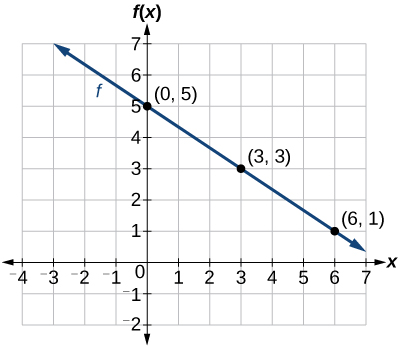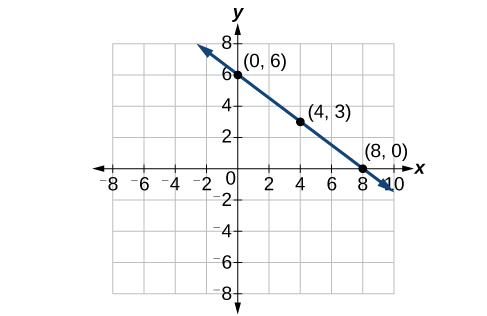# 4.1 Linear functions  (Page 7/27)

 Page 7 / 27

Is the initial value always provided in a table of values like [link] ?

No. Sometimes the initial value is provided in a table of values, but sometimes it is not. If you see an input of 0, then the initial value would be the corresponding output. If the initial value is not provided because there is no value of input on the table equal to 0, find the slope, substitute one coordinate pair and the slope into $\text{\hspace{0.17em}}f\left(x\right)=mx+b,\text{\hspace{0.17em}}$ and solve for $\text{\hspace{0.17em}}b.$

A new plant food was introduced to a young tree to test its effect on the height of the tree. [link] shows the height of the tree, in feet, $\text{\hspace{0.17em}}x\text{\hspace{0.17em}}$ months since the measurements began. Write a linear function, $\text{\hspace{0.17em}}H\left(x\right),$ where $\text{\hspace{0.17em}}x\text{\hspace{0.17em}}$ is the number of months since the start of the experiment.

 x 0 2 4 8 12 H ( x ) 12.5 13.5 14.5 16.5 18.5

$H\left(x\right)=0.5x+12.5$

## Graphing linear functions

Now that we’ve seen and interpreted graphs of linear functions, let’s take a look at how to create the graphs. There are three basic methods of graphing linear functions. The first is by plotting points and then drawing a line through the points. The second is by using the y- intercept and slope. And the third method is by using transformations of the identity function $\text{\hspace{0.17em}}f\left(x\right)=x.$

## Graphing a function by plotting points

To find points of a function, we can choose input values, evaluate the function at these input values, and calculate output values. The input values and corresponding output values form coordinate pairs. We then plot the coordinate pairs on a grid. In general, we should evaluate the function at a minimum of two inputs in order to find at least two points on the graph. For example, given the function, $\text{\hspace{0.17em}}f\left(x\right)=2x,$ we might use the input values 1 and 2. Evaluating the function for an input value of 1 yields an output value of 2, which is represented by the point $\text{\hspace{0.17em}}\left(1,2\right).\text{\hspace{0.17em}}$ Evaluating the function for an input value of 2 yields an output value of 4, which is represented by the point $\text{\hspace{0.17em}}\left(2,4\right).\text{\hspace{0.17em}}$ Choosing three points is often advisable because if all three points do not fall on the same line, we know we made an error.

Given a linear function, graph by plotting points.

1. Choose a minimum of two input values.
2. Evaluate the function at each input value.
3. Use the resulting output values to identify coordinate pairs.
4. Plot the coordinate pairs on a grid.
5. Draw a line through the points.

## Graphing by plotting points

Graph $\text{\hspace{0.17em}}f\left(x\right)=-\frac{2}{3}x+5\text{\hspace{0.17em}}$ by plotting points.

Begin by choosing input values. This function includes a fraction with a denominator of 3, so let’s choose multiples of 3 as input values. We will choose 0, 3, and 6.

Evaluate the function at each input value, and use the output value to identify coordinate pairs.

$\begin{array}{cc}\hfill x=0& \phantom{\rule{2em}{0ex}}f\left(0\right)=-\frac{2}{3}\left(0\right)+5=5⇒\left(0,5\right)\hfill \\ \hfill x=3& \phantom{\rule{2em}{0ex}}f\left(3\right)=-\frac{2}{3}\left(3\right)+5=3⇒\left(3,3\right)\hfill \\ \hfill x=6& \phantom{\rule{2em}{0ex}}f\left(6\right)=-\frac{2}{3}\left(6\right)+5=1⇒\left(6,1\right)\hfill \end{array}$

Plot the coordinate pairs and draw a line through the points. [link] represents the graph of the function $\text{\hspace{0.17em}}f\left(x\right)=-\frac{2}{3}x+5.$The graph of the linear function   f ( x ) = − 2 3 x + 5.

Graph $\text{\hspace{0.17em}}f\left(x\right)=-\frac{3}{4}x+6\text{\hspace{0.17em}}$ by plotting points.## Graphing a function using y- Intercept and slope

Another way to graph linear functions is by using specific characteristics of the function rather than plotting points. The first characteristic is its y- intercept, which is the point at which the input value is zero. To find the y- intercept, we can set $\text{\hspace{0.17em}}x=0\text{\hspace{0.17em}}$ in the equation.

bsc F. y algebra and trigonometry pepper 2
given that x= 3/5 find sin 3x
4
DB
remove any signs and collect terms of -2(8a-3b-c)
-16a+6b+2c
Will
Joeval
(x2-2x+8)-4(x2-3x+5)
sorry
Miranda
x²-2x+9-4x²+12x-20 -3x²+10x+11
Miranda
x²-2x+9-4x²+12x-20 -3x²+10x+11
Miranda
(X2-2X+8)-4(X2-3X+5)=0 ?
master
The anwser is imaginary number if you want to know The anwser of the expression you must arrange The expression and use quadratic formula To find the answer
master
The anwser is imaginary number if you want to know The anwser of the expression you must arrange The expression and use quadratic formula To find the answer
master
Y
master
master
Soo sorry (5±Root11* i)/3
master
Mukhtar
explain and give four example of hyperbolic function
What is the correct rational algebraic expression of the given "a fraction whose denominator is 10 more than the numerator y?
y/y+10
Mr
Find nth derivative of eax sin (bx + c).
Find area common to the parabola y2 = 4ax and x2 = 4ay.
Anurag
A rectangular garden is 25ft wide. if its area is 1125ft, what is the length of the garden
to find the length I divide the area by the wide wich means 1125ft/25ft=45
Miranda
thanks
Jhovie
What do you call a relation where each element in the domain is related to only one value in the range by some rules?
A banana.
Yaona
given 4cot thither +3=0and 0°<thither <180° use a sketch to determine the value of the following a)cos thither
what are you up to?
nothing up todat yet
Miranda
hi
jai
hello
jai
Miranda Drice
jai
aap konsi country se ho
jai
which language is that
Miranda
I am living in india
jai
good
Miranda
what is the formula for calculating algebraic
I think the formula for calculating algebraic is the statement of the equality of two expression stimulate by a set of addition, multiplication, soustraction, division, raising to a power and extraction of Root. U believe by having those in the equation you will be in measure to calculate it
Miranda
state and prove Cayley hamilton therom
hello
Propessor
hi
Miranda
the Cayley hamilton Theorem state if A is a square matrix and if f(x) is its characterics polynomial then f(x)=0 in another ways evey square matrix is a root of its chatacteristics polynomial.
Miranda
hi
jai
hi Miranda
jai
thanks
Propessor
welcome
jai
What is algebra
algebra is a branch of the mathematics to calculate expressions follow.
Miranda
Miranda Drice would you mind teaching me mathematics? I think you are really good at math. I'm not good at it. In fact I hate it. 😅😅😅
Jeffrey
lolll who told you I'm good at it
Miranda
something seems to wispher me to my ear that u are good at it. lol
Jeffrey
lolllll if you say so
Miranda
but seriously, Im really bad at math. And I hate it. But you see, I downloaded this app two months ago hoping to master it.
Jeffrey
which grade are you in though
Miranda
oh woww I understand
Miranda
Jeffrey
Jeffrey
Miranda
how come you finished in college and you don't like math though
Miranda
gotta practice, holmie
Steve
if you never use it you won't be able to appreciate it
Steve
I don't know why. But Im trying to like it.
Jeffrey
yes steve. you're right
Jeffrey
so you better
Miranda
what is the solution of the given equation?
which equation
Miranda
I dont know. lol
Jeffrey
Miranda
JeffreyByByBy Wey HeyBy Kimberly NicholsBy OpenStaxBy Jordon HumphreysBy OpenStaxBy Yasser IbrahimBy Saylor FoundationBy Stephen VoronByBy Mary Cohen